#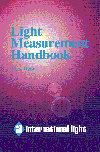Measurement Geometries

## Solid Angles

One of the key concepts to understanding the relationships between measurement geometries is that of the solid angle, or steradian.  A sphere contains 4p steradians.  A steradian is defined as the solid angle which, having its vertex at the center of the sphere, cuts off a spherical surface area equal to the square of the radius of the sphere.  For example, a one steradian section of a one meter radius sphere subtends a spherical surface area of one square meter.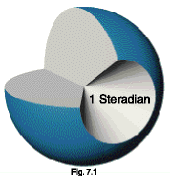The sphere shown in cross section in figure 7.1 illustrates the concept.  A cone with a solid angle of one steradian has been removed from the sphere.  This removed cone is shown in figure 7.2.  The solid angle, W, in steradians, is equal to the spherical surface area, A, divided by the square of the radius, r.

Most radiometric measurements do not require an accurate calculation of the spherical surface area to convert between units.  Flat area estimates can be substituted for spherical area when the solid angle is less than 0.03 steradians, resulting in an error of less than one percent.  This roughly translates to a distance at least 5 times greater than the largest dimension of the detector.  In general, if you follow the five times rule for approximating a point source, you can safely estimate using planar surface area.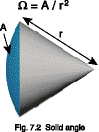Radiant flux is a measure of radiometric power.  Flux, expressed in watts, is a measure of the rate of energy flow, in joules per second.  Since photon energy is inversely proportional to wavelength, ultraviolet photons are more powerful than visible or infrared.

Luminous flux is a measure of the power of visible light.  Photopic flux, expressed in lumens, is weighted to match the responsivity of the human eye, which is most sensitive to yellow-green.  Scotopic flux is weighted to the sensitivity of the human eye in the dark adapted state.

## Units Conversion: Power

1 W (watt)
= 683.0 lm at 555 nm
= 1700.0 scotopic lm at 507 nm
1 J (joule)
= 1 W*s (watt * second)
= 107 erg
= 0.2388 gram * calories
LUMINOUS FLUX:
1 lm (lumen)
= 1.464 x 10-3 W at 555 nm
= 1/(4p) candela  (only if isotropic)
1 lm*s (lumen * seconds)
= 1 talbot (T)
= 1.464 x 10-3 joules at 555 nm

 l  nm Photopic  Luminous  Efficiency Photopic  lm / W  Conversion Scotopic  Luminous  Efficiency Scotopic  lm / W  Conversion 380  390  400  410  420  430  440  450  460  470  480  490  500  507  510  520  530  540  550  555  560  570  580  590  600  610  620  630  640  650  660  670  680  690  700  710  720  730  740  750  760  770 0.000039  .000120  .000396  .001210  .004000  .011600  .023000  .038000  .060000  .090980  .139020  .208020  .323000  .444310  .503000  .710000  .862000  .954000  .994950  1.000000  .995000  .952000  .870000  .757000  .631000  .503000  .381000  .265000  .175000  .107000  .061000  .032000  .017000  .008210  .004102  .002091  .001047  .000520  .000249  .000120  .000060  .000030 0.027  0.082  0.270  0.826  2.732  7.923  15.709  25.954  40.980  62.139  94.951  142.078  220.609  303.464  343.549  484.930  588.746  651.582  679.551  683.000  679.585  650.216  594.210  517.031  430.973  343.549  260.223  180.995  119.525  73.081  41.663  21.856  11.611  5.607  2.802  1.428  0.715  0.355  0.170  0.082  0.041  0.020 0.000589  .002209  .009290  .034840  .096600  .199800  .328100  .455000  .567000  .676000  .793000  .904000  .982000  1.000000  .997000  .935000  .811000  .650000  .481000  .402000  .328800  .207600  .121200  .065500  .033150  .015930  .007370  .003335  .001497  .000677  .000313  .000148  .000072  .000035  .000018  .000009  .000005  .000003  .000001  .000001 1.001  3.755  15.793  59.228  164.220  339.660  557.770  773.500  963.900  1149.200  1348.100  1536.800  1669.400  1700.000  1694.900  1589.500  1378.700  1105.000  817.700  683.000  558.960  352.920  206.040  111.350  56.355  27.081  12.529  5.670  2.545  1.151  0.532  0.252  0.122  .060  .030  .016  .008  .004  .002  .001

Irradiance is a measure of radiometric flux per unit area, or flux density.  Irradiance is typically expressed in W/cm2 (watts per square centimeter) or W/m2 (watts per square meter).

Illuminance is a measure of photometric flux per unit area, or visible flux density.  Illuminance is typically expressed in lux (lumens per square meter) or foot-candles (lumens per square foot).In figure 7.4, above, the lightbulb is producing 1 candela.  The candela is the base unit in light measurement, and is defined as follows:  a 1 candela light source emits 1 lumen per steradian in all directions (isotropically).  A steradian is defined as the solid angle which, having its vertex at the center of the sphere, cuts off an area equal to the square of its radius.  The number of steradians in a beam is equal to the projected area divided by the square of the distance.

So, 1 steradian has a projected area of 1 square meter at a distance of 1 meter.  Therefore, a 1 candela (1 lm/sr) light source will similarly produce 1 lumen per square foot at a distance of 1 foot, and 1 lumen per square meter at 1 meter. Note that as the beam of light projects farther from the source, it expands, becoming less dense. In fig. 7.4, for example, the light expanded from 1 lm/ft2 at 1 foot to 0.0929 lm/ft2 (1 lux) at 3.28 feet (1 m).

## Cosine Law

Irradiance measurements should be made facing the source, if possible.  The irradiance will vary with respect to the cosine of the angle between the optical axis and the normal to the detector.

## Calculating Source Distance

Lenses will distort the position of a point source.  You can solve for the virtual origin of a source by measuring irradiance at two points and solving for the offset distance, X, using the Inverse Square Law:
E1(d1 + X)2 = E2(d2 + X)2

Figure 7.5 illustrates a typical setup to determine the location of an LEDs virtual point source (which is behind the LED due to the built-in lens).  Two irradiance measurements at known distances from a reference point are all that is needed to calculate the offset to the virtual point source.## Units Conversion: Flux Density

1 W/cm2 (watts per square centimeter)
= 104 W/m2 (watts per square meter)
= 6.83 x 106 lux at 555 nm
= 14.33 gram*calories/cm2/minute

ILLUMINANCE:

1 lm/m2 (lumens per square meter)
= 1 lux (lx)
= 10-4 lm/cm2
= 10-4 phot (ph)
= 9.290 x 10-2 lm/ft2
= 9.290 x 10-2 foot-candles (fc)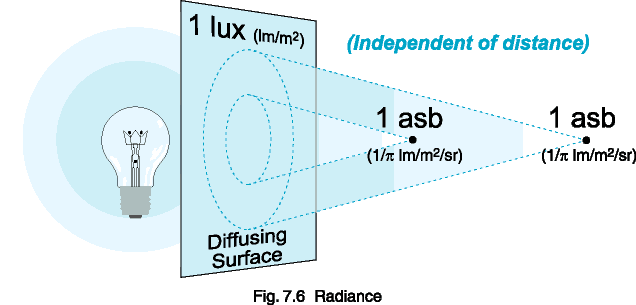Radiance is a measure of the flux density per unit solid viewing angle, expressed in W/cm2/sr.  Radiance is independent of distance for an extended area source, because the sampled area increases with distance, cancelling inverse square losses.

The radiance, L, of a diffuse (Lambertian) surface is related to the radiant exitance (flux density), M, of a surface by the relationship:

L = M / p

Some luminance units (apostilbs, lamberts, and foot-lamberts) already contain p in the denominator, allowing simpler conversion to illuminance units.

### Example:

Suppose a diffuse surface with a reflectivity, r, of 85% is exposed to an illuminance, E, of 100.0 lux (lm/m2) at the plane of the surface.  What would be the luminance, L, of that surface, in cd/m2?

### Solution:

1.) Calculate the luminous exitance of the surface:
M = E * rM = 100.0 * 0.85 =   85.0 lm/m2
2.) Calculate the luminance of the surface:
L = M / p
L = 85.0 / p = 27.1 lm/m2/sr =  27.1 cd/m2

## Irradiance From An Extended Source:

The irradiance, E, at any distance from a uniform extended area source, is related to the radiance, L, of the source by the following relationship, which depends only on the subtended central viewing angle, q, of the radiance detector:
E = p L sin2(q/2)

So, for an extended source with a radiance of 1 W/cm2/sr, and a detector with a viewing angle of 3°, the irradiance at any distance would be 2.15 x 10-3 W/cm2.  This assumes, of course, that the source extends beyond the viewing angle of the detector input optics.

## Units Conversion: Radiance & Luminance

1 W/cm2/sr (watts per sq. cm per steradian)
= 6.83 x 106 lm/m2/sr at 555 nm
= 683 cd/cm2 at 555 nm

LUMINANCE:

1 lm/m2/sr (lumens per sq. cm per steradian)
= 1 candela/m2 (cd/m2)
= 1 nit
= 10-4 lm/cm2/sr
= 10-4 cd/cm2
= 10-4 stilb (sb)
= 9.290 x 10-2 cd/ft2
= 9.290 x 10-2 lm/ft2/sr
= p apostilbs (asb)
= p cd/p/m2
= p x 10-4 lamberts (L)
= p x 10-4 cd /p/cm2
= 2.919 x 10-1 foot-lamberts (fL)
= 2.919 x 10-1 lm/p/ft2/sr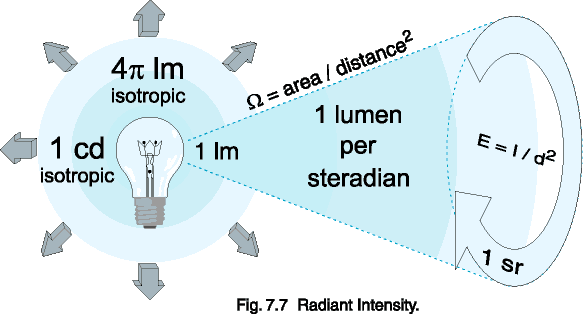Radiant Intensity is a measure of radiometric power per unit solid angle, expressed in watts per steradian.  Similarly, luminous intensity is a measure of visible power per solid angle, expressed in candela (lumens per steradian).  Intensity is related to irradiance by the inverse square law, shown below in an alternate form:
I = E * d2

If you are wondering how the units cancel to get flux/sr from flux/area times distance squared, remember that steradians are a dimensionless quantity.  The solid angle equals the area divided by the square of the radius, so d2=A/W, and substitution yields:

I = E * A / W

The biggest source of confusion regarding intensity measurements involves the difference between Mean Spherical Candela and Beam Candela, both of which use the candela unit (lumens per steradian).  Mean spherical measurements are made in an integrating sphere, and represent the total output in lumens divided by 4p sr in a sphere.  Thus, a one candela isotropic lamp produces one lumen per steradian.Beam candela, on the other hand, samples a very narrow angle and is only representative of the lumens per steradian at the peak intensity of the beam.  This measurement is frequently misleading, since the sampling angle need not be defined.

Suppose that two LEDs each emit 0.1 lm total in a narrow beam: One has a  10° solid angle and the other a 5° angle.  The 10° LED has an intensity of 4.2 cd, and the 5° LED an intensity of 16.7 cd.  They both output the same total amount of light, however -- 0.1 lm.

A flashlight with a million candela beam sounds very bright, but if its beam is only as wide as a laser beam, then it wont be of much use.  Be wary of specifications given in beam candela, because they often misrepresent the total output power of a lamp.

## Units Conversion: Intensity

= 12.566 watts (isotropic)
= 4*p W
= 683 candela at 555 nm

LUMINOUS INTENSITY:

= 1 candela (cd)
= 4*p lumens (isotropic)
= 1.464 x 10-3 watts/sr at 555 nm

## Converting Between Geometries

Converting between geometry-based measurement units is difficult, and should only be attempted when it is impossible to measure in the actual desired units.  You must be aware of what each of the measurement geometries implicitly assumes before you can convert.  The example below shows the conversion between lux (lumens per square meter) and lumens.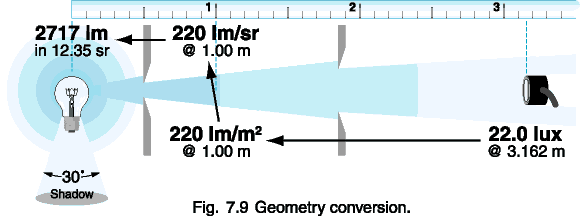### Example:

You measure 22.0 lux from a light bulb at a distance of 3.162 meters.  How much light, in lumens, is the bulb producing?  Assume that the clear enveloped lamp is an isotropic point source, with the exception that the base blocks a 30° solid angle.

### Solution:

1.) Calculate the irradiance at 1.0 meter:
E1 = (d2 / d1)2 * E2
E1.0 m = (3.162 / 1.0)2 * 22.0 = 220 lm/m2
2.) Convert from lm/m2 to lm/sr at 1.0 m:
220 lm/m2 * 1 m2/sr =  220 lm/sr
3.) Calculate the solid angle of the lamp:
W = A / r2 = 2ph / r = 2p[1 - cos(a / 2)]
W = 2p[1 - cos(330 / 2)] = 12.35 sr
4.) Calculate the total lumen output:
220 lm/sr * 12.35 sr = 2717 lm

## Next

### Contents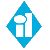HOME